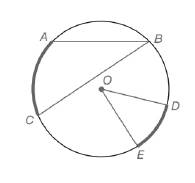Chapter 6.1, Problem 8E### Elementary Geometry for College St...

6th Edition
Daniel C. Alexander + 1 other
ISBN: 9781285195698

#### Solutions

Chapter
Section### Elementary Geometry for College St...

6th Edition
Daniel C. Alexander + 1 other
ISBN: 9781285195698
Textbook Problem
1 views

# For Exercises 1 to 8, use the figure provided.Exercises 1-8If m E C A B D ⌢ = 312 ∘ , find m ∠ D O E .

To determine

To calculate:

The mDOE, if mECABD=312 by using the provided figure.

Explanation

Given:

The provided figure is

Postulate used:

Central angle postulate:

In a circle, the degree measure of a central angle is equal to the degree measure of its intercepted arc.

Calculation:

The intercepted arc of DOE is DE.

From the figure, we see that the arcs DE,ECABD form the whole circle.

Therefore mDE+mECABD=360

Substitute mECABD=312 in mDE+mECABD=360

### Still sussing out bartleby?

Check out a sample textbook solution.

See a sample solution

#### The Solution to Your Study Problems

Bartleby provides explanations to thousands of textbook problems written by our experts, many with advanced degrees!

Get Started

#### Find more solutions based on key concepts